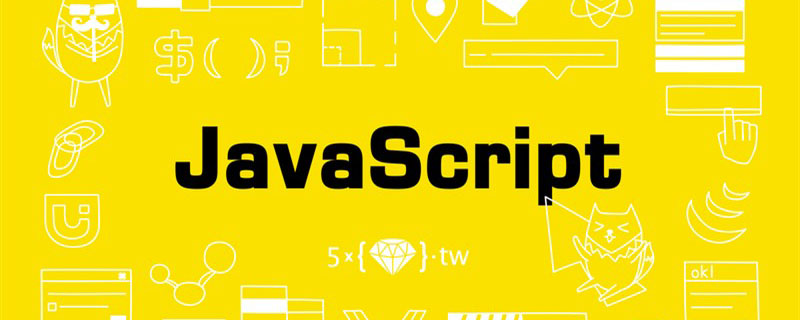>
>
>

# Javascript对象创建模式

##### 云起科技：2021-01-25 16:17:45    阅读数：302

Javascript有多种创建对象的方式，新手和老手都可能感到不知所措，不确定应该使用哪种方式。文本将介绍常见的对象常见模式和最佳的实践。### 对象字面量：Object Literals

``````var o = {
x: 42,
y: 3.14,
f: function() {},
g: function() {}
};``````

### 工厂模式：Factory Functions

``````function thing() {
return {
x: 42,
y: 3.14,
f: function() {},
g: function() {}
};
}
var o = thing();``````

### 构造函数模式

``````function Student(name,age){
this.name=name;
this.age=age;
this.myName=function(){
};
}
var student1_ = new Student('aaa',15);
var student2_ = new Student('bbb',18);``````

### 原型链：Prototype Chains

Javascript提供了一种内置的在对象之间共享数据的机制，称为原型链。当访问对象的属性时，它可以通过委托给其他对象来满足该请求。可以利用这一点来修改工厂函数，使它创建的每个对象只包含自己特有的数据，而对其他属性的请求则全部委托给原型链上共有的一个对象：

``````var thingPrototype = {
f: function() {},
g: function() {}
};

function thing() {
var o = Object.create(thingPrototype);

o.x = 42;
o.y = 3.14;

return o;
}

var o = thing();``````

``````thing.prototype.f = function() {};
thing.prototype.g = function() {};

function thing() {
var o = Object.create(thing.prototype);

o.x = 42;
o.y = 3.14;

return o;
}

var o = thing();``````

### ES5类：ES5 Classes

``````function create(fn) {
var o = Object.create(fn.prototype);

fn.call(o);

return o;
}

// ...

Thing.prototype.f = function() {};
Thing.prototype.g = function() {};

function Thing() {
this.x = 42;
this.y = 3.14;
}

var o = create(Thing);``````

``````Thing.prototype.f = function() {};
Thing.prototype.g = function() {};

function Thing() {
this.x = 42;
this.y = 3.14;
}

var o = new Thing();``````

### ES6类：ES6 Classes

``````class Thing {
constructor() {
this.x = 42;
this.y = 3.14;
}

f() {}
g() {}
}

const o = new Thing();``````

### 特点

• 工厂模式通过闭包实现
• 类通过weak maps实现